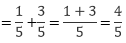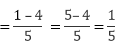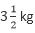# RD Sharma Class 6 ex 6.8 Solutions Chapter 6 Fractions

In this chapter, we provide RD Sharma Class 6 ex 6.8 Solutions Chapter 6 Fractions for English medium students, Which will very helpful for every student in their exams. Students can download the latest RD Sharma Class 6 ex 6.8 Solutions Chapter 6 Fractions Maths pdf, Now you will get step by step solution to each question.

# Chapter 6: Fractions – Exercise 6.8

### Question: 1

Write these fractions appropriately as additions or subtraction:

Solve:

### Solution:

i) The given fractions are:

ii) The given fractions are:

iii) the given fractions are:

iv) the given fractions are:

v) The given fractions are:

vi) the given fractions are:

vii) the given fractions are:

viii) the given fractions are:

Hence the answer is 1/1 = 1

ix) the given fractions are:

Hence the answer is 5/1 = 5

x) the given fractions are:

xi) the given fractions are:

### Question: 3

Shikha painted 1/5 of the wall space in her room. Her brother ravish helped and painted 3/5 of the wall space. How much did they paint together? How much the room is left unpainted?

### Solution:

Shikha painted 1/5 of the wall space in her room

Ravish painted 3/5 of the wall space

Wall space painted by both of them togetherUnpainted part of the room### Question: 4

Ramesh boughtsugar whereas rohit boughtof sugar. Find the total amount of sugar bought by both of them.

### Solution:

Quantity of sugar bought by ramesh =Quantity of sugar bought by rohit =Total amount of sugar bought by them:

Quantity of sugar bought by rohit + Quantity of sugar bought by ramesh

= 6 kg (Dividing numerator and denominator by their HCF (6))

### Question: 5

The teacher taught 3/5 of the book, Vivek revised 1/5 more on his own. How much does he still have to revise?

### Solution:

Fraction of the book taught by the teacher = 3/5

Fraction of the book revised by vivek = 1/5

Fraction of the book still left for revision by vivek:

Therefore, Fraction of the book still left for revision by vivek is 2/5

### Question: 6

Amit was given 5/7 of a bucket of oranges. What fraction of oranges was left in the basket?

### Solution:

Fraction of oranges given to amit = 5/7

Fraction of oranges left in the basket:

Therefore, Fraction of oranges left in the basket is 2/7

### Solution:

i) Given:

Therefore,

ii) Given:

Therefore,

iii) Given:

Therefore,

iv) Given:

Therefore,

All Chapter RD Sharma Solutions For Class 6 Maths

I think you got complete solutions for this chapter. If You have any queries regarding this chapter, please comment on the below section our subject teacher will answer you. We tried our best to give complete solutions so you got good